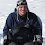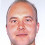# Learning Clojure

## Monday, September 6, 2010

### reduce-seq: An Exercise in Laziness and Futility

```;; A futile design process

;; The other day, I found myself wanting a running average of an infinite sequence

;; Here is an example of such a sequence:
(def integers (iterate inc 0))

;; At first, I took the easy way out by just calculating it
(defn runavg [sq]
(fn [n]
(/ (reduce + (take (inc n) sq))
(inc n))))

;; So here is the fifth running average
((runavg integers) 5)

;; After a while, I had occasion to make a list of the running averages themselves
(def runavgs (map (runavg integers) (iterate inc 0)))

(take 5 runavgs)

;; this list proved very useful, but Schlemiel the Painter is at work here:

(time (count (take 100    (map (runavg integers) (iterate inc 0))))) ;;12ms
(time (count (take 1000   (map (runavg integers) (iterate inc 0))))) ;;1200ms
;; (time (count (take 10000 (map (runavg integers) (iterate inc 0))))) ;;120s
;; (time (count (take 100000 (map (runavg integers) (iterate inc 0))))) ;;3 hours

;; This wasn't a problem for my program as first written, but as I pushed it
;; further this became a major bottleneck, and so I decided to optimize the
;; running average to only look at each element once.
(defn runavg
([lst] (runavg 0 0 lst))
([sum count lst]
(if (empty? lst) '()
(let [newsum (+ sum (first lst))
newcount (inc count)]
(cons (/ newsum newcount)
(runavg newsum newcount (rest lst)))))))

(runavg '(1 2 3 4 5))

;; Of course, we can make this work for infinite sequences too just by wrapping
;; the function with lazy-seq
(defn runavg
([lst] (runavg 0 0 lst))
([sum count lst]
(lazy-seq
(if (empty? lst) '()
(let [newsum (+ sum (first lst))
newcount (inc count)]
(cons (/ newsum newcount)
(runavg newsum newcount (rest lst))))))))

(take 5 (runavg integers))

;; Performance, predictably is vastly improved.
(time (count (take 100    (runavg integers))))) ;;0.3ms
(time (count (take 1000   (runavg integers))))) ;;3ms
(time (count (take 10000  (runavg integers))))) ;;30ms
(time (count (take 100000 (runavg integers))))) ;;300ms

;; Now, in this new algorithm, we have an accumulator, which stores the sum of
;; the elements so far And another which stores the count so far, and this
;; automatically made me think of reduce:
(reduce + (range 1 10))                       ;;summing with reduce
(reduce (fn[a x] (inc a)) (range 1 10))       ;;counting with reduce

;; Of course we can combine these two reductions
(reduce (fn [[sum count] x] [(+ sum x) (inc count)]) [0 0] (range 1 10))

;; But if we want a running average, as above, then we'd really like to see the
;; state of the accumulator at every step, and also, reduce will blow up on an
;; infinite sequence.

;; And so it occurred to me that I could write a lazy version of reduce, which I called
;; reduce-seq

(defn reduce-seq [f acc sq]
(if (empty? sq) (list acc)
(let [next-acc (f acc (first sq))]
(cons acc (reduce-seq f next-acc (rest sq))))))

;; Now, it's a simple matter to lazy this up:
(defn reduce-seq [f acc sq]
(lazy-seq
(if (empty? sq) (list acc)
(let [next-acc (f acc (first sq))]
(cons acc (reduce-seq f next-acc (rest sq)))))))

;; Here are some trivial reductions
(reduce-seq + 0 '(0 1 2 3 4 5))             ;; (0 1 3 6 10 15)
(take 5 (reduce-seq + 0 integers)))         ;; (0 0 1 3 6)
(take 5 (reduce-seq * 1 (drop 1 integers))) ;; (1 1 2 6 24)

;; A new way to define the factorials is always pleasing, and the laziness makes
;; it pretty efficient!

;; Here's it working with the function and accumulator above
(reduce-seq
(fn [[sum count] x] [(+ sum x) (inc count)])
[0 0]
(range 5)) ;;([0 0] [0 1] [1 2] [3 3] [6 4] [10 5])

;; And here's how we could use it to define runavg
(defn running-average [sq]
(map #(apply / %)
(drop 1
(reduce-seq
(fn [[sum count] x] [(+ sum x) (inc count)])
[0 0]
sq))))

(running-average (range 5))          ;; (0 1/2 1 3/2 2)
(take 10 (running-average integers)) ;; (0 1/2 1 3/2 2 5/2 3 7/2 4 9/2)

;; So at this point, I was starting to feel pretty smug.
;; A new version of factorial, and a functional abstraction like map, filter, and reduce that
;; seemed to be more general than any of them, and adapted for infinite sequences besides.
;; I'd never seen anything like that before.

;; And it occurred to me that if I was going to generalize reduce, I'd better deal with
;; the case where the first value of the sequence is used as the initial accumulator too:
(defn reduce-seq
([f acc sq]
(lazy-seq
(if (empty? sq) (list acc)
(let [next-acc (f acc (first sq))]
(cons acc (reduce-seq f next-acc (rest sq)))))))
([f sq] (reduce-seq f (first sq) (rest sq))))

;; reduce-seq should work as much like reduce as possible

(reduce     + 0 '(1 2 3 4 5))     ;; 15
(reduce-seq + 0 '(1 2 3 4 5))     ;; (0 1 3 6 10 15)
(reduce     +   '(1 2 3 4 5))     ;; 15
(reduce-seq +   '(1 2 3 4 5))     ;; (1 3 6 10 15)
(reduce + 0 (range 4)) ;;6
(take 5 (reduce-seq + 0 (iterate inc 0))) ;; (0 0 1 3 6)
(reduce + 0 '())     ;; 0
(reduce-seq + 0 '()) ;; (0)

;; So far so good, but I've violated the principle of least surprise here:
(reduce + '())     ;; 0
(reduce-seq + '()) ;; (nil)

;; Here's a regression test that will allow me to mess about with the function
;; while preserving its good behaviours
(defn test-reduce-seq [reduce-seq]
(cond (not= (reduce-seq + 0 '(1 2 3 4 5)) '(0 1 3 6 10 15)) "fail"
(not= (reduce-seq +   '(1 2 3 4 5)) '(1 3 6 10 15))    "fail"
(not= (take 5 (reduce-seq + 0 (iterate inc 0))) '(0 0 1 3 6)) "fail"
(not= (reduce-seq + 0 '()) '(0)) "fail"
(not= (reduce-seq +   '(1))   '(1)) "fail"
(not= (reduce-seq + 1 '(1))    '(1 2)) "fail"
(not= (nth (reduce-seq + (iterate inc 0)) 10000) (* 1/2 10000 10001)) "fail"
(not= (reduce-seq #(assoc %1 %2 (inc (get %1 %2 0))) {} "aab")
'({} {\a 1} {\a 2} {\b 1, \a 2})) "fail"
(not= (reduce-seq + '()) '(0)) "fix me!!"
:else "pass"))

(test-reduce-seq reduce-seq)

;; We can slightly shorten the function by noticing that we add the accumulator to
;; the sequence whether the sequence is empty or not.
(defn reduce-seq
([f acc sq]
(lazy-seq
(cons acc
(if (empty? sq) '()
(let [next-acc (f acc (first sq))]
(reduce-seq f next-acc (rest sq)))))))
([f sq] (reduce-seq f (first sq) (rest sq))))

(test-reduce-seq reduce-seq)

;; It's for some reason considered better style with the lazy functions to use when-let and seq
;; instead of if and empty?

;; If we swap the arms of the if statement:
(defn reduce-seq
([f acc sq]
(lazy-seq
(cons acc
(if-let [sq (seq sq)]
(let [next-acc (f acc (first sq))]
(reduce-seq f next-acc (rest sq)))
'()))))
([f sq] (reduce-seq f (first sq) (rest sq))))

(test-reduce-seq reduce-seq)

;; and then notice that (cons a (if-let b c '()))
;; is equivalent to     (cons a (when-let b c))
;; then we can shorten the function yet further, and put it into this 'house style'
(defn reduce-seq
([f acc sq]
(lazy-seq
(cons acc
(when-let [sq (seq sq)]
(reduce-seq f (f acc (first sq)) (rest sq))))))
([f sq] (reduce-seq f (first sq) (rest sq))))

(test-reduce-seq reduce-seq)

;; This actually looks a bit the wrong way round to me, but it's shorter than
;; the original, so it's probably just a matter of getting used to this style in
;; the same way that I'm used to the SICP style of putting the empty case first.

;; Having put it into the house style, we can fix the bug with the empty list
;; and no accumulator:
(defn reduce-seq
([f acc sq]
(cons acc
(lazy-seq
(when-let [sq (seq sq)]
(reduce-seq f (f acc (first sq)) (rest sq))))))
([f sq] (if-let [sq (seq sq)]
(reduce-seq f (first sq) (rest sq))
(list (f)))))

(test-reduce-seq reduce-seq)

;; And as a final flourish, I thought it would be nice to use the destructuring notation
(defn reduce-seq
([f acc sq]
(cons acc
(lazy-seq
(when-let [[head & tail] (seq sq)]
(reduce-seq f (f acc head) tail)))))
([f sq] (if-let [[head & tail] (seq sq)]
(list (f)))))

(test-reduce-seq reduce-seq)

;; That works fine, but actually, the function look more 'noisy' like that than before,
;; so I prefer the penultimate version.

;; Feeling pretty happy with this, I thought about it for a bit, and decided that
;; "reductions" would be a better name for reduce-seq

;; So my final version was:
(defn reductions
([f acc sq]
(cons acc
(lazy-seq
(when-let [sq (seq sq)]
(reductions f (f acc (first sq)) (rest sq))))))
([f sq] (if-let [sq (seq sq)]
(reductions f (first sq) (rest sq))
(list (f)))))

"WARNING: reductions already refers to: #'clojure.core/reductions in namespace: user, being replaced by: #'user/reductions"

;; Here's the source of the standard version, which was apparently added in version 1.2:

(defn reductions
"Returns a lazy seq of the intermediate values of the reduction (as
per reduce) of coll by f, starting with init."
([f coll]
(lazy-seq
(if-let [s (seq coll)]
(reductions f (first s) (rest s))
(list (f)))))
([f init coll]
(cons init
(lazy-seq
(when-let [s (seq coll)]
(reductions f (f init (first s)) (rest s)))))))

;; I suppose that there are some positives to take away from this:

;; At least I know that I am thinking along roughly the same lines as the designers
;; of Clojure....

;;;;;;;;;;;;;;;;;;;;;;;;;;;;;;;;;;;;;;;;;;;;;;;;;;;;;;;;;;;;;;;;;;;;;;;;;;;;;;;;;;;;;
;; Footnote

;; Why doesn't this work any more?
;; (use 'clojure.contrib.trace)
;; (dotrace (reduce-seq) (take 5 (reduce-seq + 0 '(1 2 3 4 5))))

```

1.(running-average (range 5)) ;; (0 1/2 1 3/2 2)
(map #(/ % 2) (range 5)) ;; (0 1/2 1 3/2 2)

;;i think you actually want
(running-average (range 5)) ;; (0 1/2 2/3 3/4 4/5)

maybe i'm misunderstanding what you want out of your running average but to me a running average would be the average of all numbers in the sequence up to the nth number.

2.Hi Brandon, thanks for the comment!

I was trying to calculate something like this:

sequence : 0 1 2 3 4 5 6
running sum : 0 1 3 6 10 15 21
element count : 1 2 3 4 5 6 7
quotients : 0 1/2 3/3 6/4 10/5 15/6 21/7
simplified : 0 1/2 1 3/2 2 5/2 3

(It's roughly the area of a triangle over the base of the triangle, x->1/2x*x/x)

How did you get yours?

3.whoops. i messed up my logic. i guess i was just confused that the running average of integers is simply n/2

4.That's crazy, your final function turned out to be identical to the version in Clojure. Just a couple different var names and your signatures are in reverse order - everything else is the same.

Given how flexible Clojure is, it's interesting that there's a clearly idiomatic way that emerges.